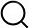# Also in the Article

2.4. Standard approach for QRS-Ta estimation

Procedure

The origin of both the QRS and T-wave loops for the identification of the QRS and T-wave vectors is taken at $v→0=v→(t0)$, where $t0=QRSON$. The QRS and T-wave loops are then defined by vectors:

The peak vectors $v→QRSmax$ and $v→TWmax$ are defined as those associated with the largest magnitude, i.e. $v→QRSmax=v→QRStm$, with $tm=arg(max(v→QRS(t)))$, and $v→TWmax=v→TWtm$, with $tm=arg(max(v→TW(t)))$. The mean vectors $v→QRSmean$ and $v→TWmean$ are measured as the temporal mean of $v→QRSt$ and $v→TWt$. The spatial peak QRS-Ta, ($αP$), is measured as the angle between $v→QRSmax$ and $v→TWmax$ while the spatial mean QRS-Ta, ($αM$), is measured as the angle between $v→QRSmean$ and $v→TWmean$.

Q&A